#### Chapter 8 Lines and Angle R.D. Sharma Solutions for Class 9th Math Exercise 8.3

Exercise 8.3

1. In Fig. 8.56, l1 and l2 intersect at O, forming angles shown in the figure. If x = 45, find the values of y, z and u.

Solution

Given that,
x = 45°, y =?, Z = ?, u=?
Vertically opposite angles are equal
Therefore, z = x = 45
z and u are angles that are a linear pair
Therefore, z + u = 180°
z = 180 – u
⇒ u = 180 – x
⇒ u = 180 – 45
⇒ u = 135°
x and y angles are a linear pair.
x+ y = 180°
⇒ y = 180 – x
⇒ y =180 – 45
⇒ y = 135°

Hence, x = 45°, y = 135°, z = 135° and u = 45°

2. In Fig. 8.57, three coplanar lines intersect at a point O, forming angles as shown in the figure. Find the values of x, y, z and u.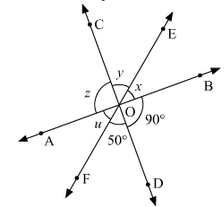Solution

Vertically opposite angles are equal
So, ∠SOD = z = 90° ∠DOF = y = 50°
Now, x + y + z = 180 [Linear pair]
x + y + z = 180
⇒ 90 + 50 + x = 180
⇒ x = 180 – 140
⇒ x = 40
Hence, values of x, y, z and u are 40, 50, 90 and 40 respectively in degrees.

3. In Fig. 8.58, find the values of x, y and z.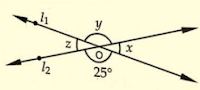Solution
From the given figure,
y = 25 [Vertically opposite angles are equal]
Now ∠x+∠y=180° [Linear pair of angles]
x = 180 – 25
⇒ x = 155°
Also,
z = x = 155° [Vertically opposite angles]
y = 25°
z = 155°

4. In Fig. 8.59, find the value of x.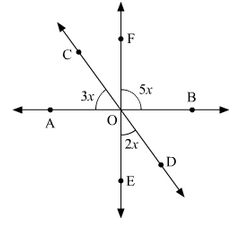Solution

In the following figure we have to find the value of x.
Vertically opposite angles are equal
AOE = BOF = 5x
Linear pair ∠COA+∠AOE+∠EOD=180°
3x + 5x + 2x = 180°
⇒ 10x = 180
⇒ x = 180/10
⇒ x = 18
Hence, the value of x = 18°

5. Prove that the bisectors of a pair of vertically opposite angles are in the same straight line.

Solution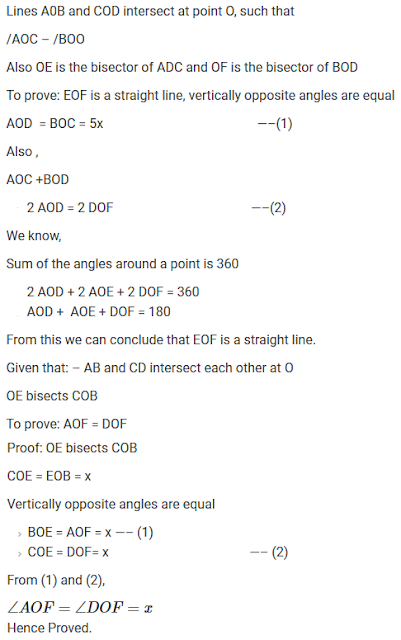6. If two straight lines intersect each other, prove that the ray opposite to the bisector of one of the angles thus formed bisects the vertically opposite angle.

Solution

Let AB and CD intersect at a point O
Also, let us draw the bisector OP of ∠AOC
Therefore,
∠AOP = ∠POC - (i)
Also, let’s extend OP to Q.
We need to show that, OQ bisects BOD
Let us assume that OQ bisects BOD , now we shall prove that POQ is a line.
We know that,
∠AOC and ∠DOB are vertically opposite angles. Therefore, these must be equal,
That is ∠AOC = ∠DOB —(ii)
∠AOP and ∠BOQ are vertically opposite angles.
Therefore, ∠AOP = ∠BOQ

Similarly, ∠POC = ∠DOQ

We know that : AOP + AOD + DOQ + POC + BOC + BOQ = 360°
2 ∠AOP + ∠AOD +2 ∠DOQ+ ∠BOC =360°
2 ∠AOP + 2 ∠AOD + 2 ∠DOQ = 360°
2(∠AOP + ∠AOD + ∠DOQ) = 360°
∠AOP + ∠AOD + ∠DOQ = 360 /2
∠AOP + ∠AOD + ∠DOQ = 180°
Thus, ∠POQ is a straight line.
Hence, our assumption is correct.
Therefore, we can say that if the two straight lines intersect each other, then the ray opposite to the bisector of one of the angles thus formed bisects the vertically opposite angles.

7. If one of the four angles formed by two intersecting lines is a right angle, then show that each of the four angles is a right angle.

SolutionGiven,
AB and CD are two lines intersecting at O, such that ∠BOC=90°, ∠AOC=90°, ∠AOD=90° and ∠BOD = 90°.

Proof :
Given that B0C = 90°
Vertically opposite angles are equal
∠BOC = ∠AOD = 90°
AOC, BOC are a Linear pair of angles
∠AOC+∠BOC=180° [Linear pair]
∠AOC +90° = 180°
∠AOC = 90°

Therefore, ∠AOC = ∠BOD = 90°  (Vertically opposite angles)
Hence, ∠AOC = ∠BOC = ∠BOD = ∠AOD = 90°

8. In Fig. 8.60, rays AB and CD intersect at O.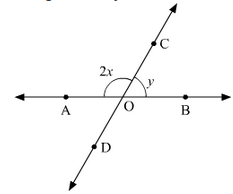(i) Determine y when x = 60°
(ii) Determine x when y = 40°

Solution

(i) Given x = 60°
∠AOC, ∠BOC are linear pair of angles ∠AOC+∠BOC=180°
⇒ 2x + y = 180°
⇒ 2×60 + y = 180° [since x = 60°]
⇒ y = 60°

(ii) Given y = 40°
∠AOC and ∠BOC are linear pair of angles ∠AOC+∠BOC=180°
⇒ 2x + y = 180
⇒ 2x + 40 = 180
⇒ 2x =180 – 140
⇒ 2x = 140
⇒ x = 70°

9. In Fig 8.61, lines AB, CD and EF intersect at O, Find the measure of ∠AOC, ∠COF, ∠DOE and ∠BOF.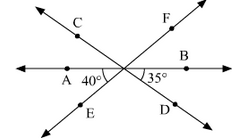Solution

AOE and EOB are linear pair of angles ∠AOE+∠EOB=180°
∠AOE+∠DOE+∠BOD=180°
⇒ DOE = 180 – 40 – 35 = 105°
Vertically opposite side angles are equal.
∠DOE = ∠COF =105°
Now, ∠AOE+∠AOF=180° [Linear pair]
∠AOE + ∠AOC + ∠COF = 180°
⇒ 40 + AOC +105 = 180°
⇒ AOC = 180 – 145
⇒ AOC = 35°
Also, BOF = AOE = 40° (Vertically opposite angles are equal)

10. AB, CD and EF are three concurrent lines passing through the point O such that OF bisects ∠BOD. If ∠BOF = 35°, find ∠BOC and ∠AOD.

Solution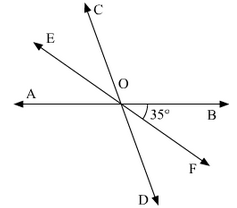Given,
OF bisects BOD
∠BOF = 35°
Angles ∠BOC and ∠AOD are unknown.
∠BOD = 2 ∠BOF = 70° [since BOD is bisected]
∠BOD = ∠AOC = 70° [ BOD and AOC are vertically opposite angles]
Now,
∠BOC + ∠AOC = 180°
⇒ ∠BOC +70 = 180°
⇒ ∠BOC =110°
⇒ ∠AOD = ∠BOC = 110° (Vertically opposite angles)

11. In Fig. 8.62, lines AB and CD intersect at O. If ∠AOC + ∠BOE = 70° and ∠BOD = 40°, find ∠BOE and reflex ∠COE.Solution

Given that,
∠AOC + ∠BOE = 70 and ∠BOD = 40
To find ∠BOE,
Here, ∠BOD and ∠AOC an vertically opposite angles
∠BOD = ∠AOC = 40
Given, ∠AOC+∠BOE=70°
⇒ 40 + ∠BOF = 70°
⇒ ∠BOF = 70° – 40°
⇒ ∠BOE = 30°
∠AOC and ∠BOC are lines pair of angles
⇒ ∠AOC + ∠COF + ∠BOE = 180°
⇒ ∠COE = 180° – 30° – 40°
⇒ ∠COE = 110°
Hence, Reflex ∠COE = 360° – 110° – 250°.

12. Which of the following statements are true (T) and which are false (F) ?
(i) Angles forming a linear pair are supplementary.
(ii) If two adjacent angles are equal, then each angle measures 90°.
(iii) Angles forming a linear pair can both be acute angles.
(iv) If angles forming a linear pair are equal, then each of these angles is of measure 90°.

Solution

(i) True
(ii) False
(iii) False
(iv) True

13. Fill in the blanks so as to make the following statements true:-
(i) If one angle of a linear pair is acute, then its other angle will be ........
(ii) A ray stands on a line, then the sum of the two adjacent angles so formed is ..........
(iii) If the sum of two adjacent angles is 180°, then the ........ arms of the two angles are opposite rays.

Solution

(i) Obtuse angle
(ii) 180 degrees
(iii) Uncommon